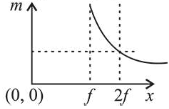# An object is gradually moving away from the focal point of a concave mirror along the axis of the mirror.Question:

An object is gradually moving away from the focal point of a concave mirror along the axis of the mirror. The graphical representation of the magnitude of linear magnification $(m)$ versus distance of the object from the mirror $(x)$ is correctly given by

(Graphs are drawn schematically and are not to scale)

1.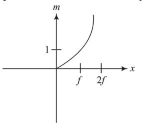2.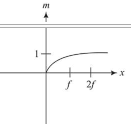3.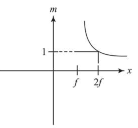4.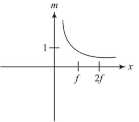Correct Option: 3

Solution:

(3) Using mirror formula, magnification is given by

$m=\frac{f}{u-f}=\frac{-1}{1-\frac{u}{f}}$

At focus magnification is $\infty$

And at $u=2 f$, magnification is 1 .

Hence graph (4) correctly depicts ' $m$ ' versus distance

of object ' $x$ ' graph.